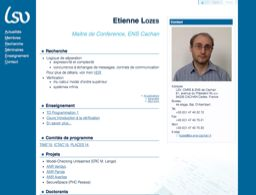# Higher Order Fixpoint Logic: Expressiveness and Descriptive Complexity27/03/2015
Intervenant(s) : Etienne Lozes
Process equivalence checking and model-checking are two different ways of verifying systems. In the case of model-checking, the modal mu-calculus plays the role of an assembly language, so that any model-checker for the modal mu-calculus yields a model-checker for any other standard temporal logic. The situation is rather different with process equivalences: there is in general no obvious way of reducing a process equivalence to another one.
We present a logical framework based on an expressive modal fixpoint logic which is capable of defining many process equivalence relations. For each such equivalence there is a fixed formula which is satisfied by a pair of processes if and only if they are equivalent with respect to this relation. We explain how to do model checking for this logic. This allows model checking technology to be used for process equivalence checking.
The first ingredient of our logic is polyadicity: a formula describes a relation among states of the system(whereas it describes a property of states in the monadic case). Martin Otto showed that the polyadic modal mu-calculus captures the class P[bis] of problems that are bisimulation invariant and can be decided in polynomial time: all problems expressed in this logic are in this class, and vice versa. For instance, bisimulation can be expressed in the polyadic modal mu-calculus, but trace equivalence can’t (unless PTIME=PSPACE).
The second ingredient of our logic is higher-orderness. Following a proposal of Viswanathan, we introduce the ability to define predicate transformers, transformers of predicate transformers, etc. and we allow them to be recursively defined like standard predicates of the modal mu-calculus. This feature is essential for expressing linear-time process equivalences (often PSPACE complete). We extend Martin Otto’s result to our logic, and show that our logic, at the order 1, captures EXPTIME[bis]. We moreover introduce a syntactic condition reminiscent to tail recursion in functional programming that all our formulas defining process equivalences satisfy. We show that under this restriction, our logic captures PSPACE[bis] at order 1 and NLOGSPACE[bis] at order 0.